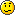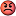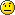# Maths Quiz

Status
Not open for further replies.

Why not?

#### Kl@w-24

##### Slideshow Bob
Hmm... Is this one valid?

-1 = √-1 * √-1#### dreamcatcher

##### Rockin g33k
i^2=i*i

here both sides should cancel out. The same way we can prove 1=2, 1=4, blah balh. Its not feasible.

#### srinivasdevulapally

##### The Masterminded
4x+2y=100
x+y=30

x=y-30
4(y-30)+2y=100

120-2y=100
y=10

x+10=30

x=20

So 20 cows and 10 hens

Dont just go for equations, just use ur brainFor cows or hens, there will be 2 legs in common. There are 100 legs in total and there are 30 in number. Hence 30 x 2 = 60 legs are common.

But the extra 40 (100 - 60) legs are nothing but cows' legs.

Since each cow has 2 extra legs,

40/2 = 20 cows

rest (30-20) 10 are hens...That's all !!!-1 = √1
-1 = 1Why does this happen?√1 can be (-1)^2 or (1)^2 so u cannot say that √1 is 1Last edited:

#### Krazzy Warrior

##### "Aal Izz Well"
Its takes time to understand if u don't get the logic but very simple if u are through

1!= 1
2!= 2
3!= 6
4!= 24
5!= 120

From 5! every no. ends with a zero...
Any no ending with zero raised to any power gives a number which has the last digit as zero.

So all the other no.s from 5! onwards will end in zero so we should calculate only the last digit for the following

(1!)^(1!) + (2!)^(2!) + (3!)^(3!) +(4!)^(4!)

(1!)^(1!) = 1

(2!)^(2!) = 2^2 = 4

(3!)^(3!) = 6^6 =... ends with 6 (since 6 raised to any power gives a no. whose last digit is always 6

(4!)^(4!) = 24^24 = 4^24 * 6^24 =....ends with 6

{since 6^24 ends with 6 and

4 raised to the odd power gives no. ending wit 4
4 raised to the even power gives no. ending with 6
eg:
4^1 = 4
4^2 = 16
4^3 = 64
4^4 = 256 and so on...
4^24 ends with 6

6*6 ends with 6}

hence the last digit will be the addition of these last digits
1+4+6+6 = 17 hence ends with 7

I more trick(i got that last night):-

(1)^1 + (2)^2.....+(n)^n --->the unit digit will be same as 1+2+.....+n...and to find 1+2+.....+n we apply this formula :- n*(n+1)/2

so for ur question 100*(100+1)/2--> 100*101/2 = 5050 the last digit is zero..therefor the unit digit of (1!)^(1!)....+(100!)^(100!) will be zero....

A question (just u have to explain..you will be shock):-

Three friends went to a restaurant and had a bill of Rs. 30...each decided to pay Rs.10...the owner was impressed by the waiter who served this friend and return Rs. 10 to the waiter telling him to return Rs. 2 to each person (2*3=6) and to keep Rs. 4 with u (waiter).. (6+4=10)...As Rs. 2 is returned so each person paid Rs.8 (10-2) so 8*3=24 (all three together) and Rs. 4 from waiter make 24+4=28 ..Where is this Rs. 2 gone ?Prove that 0.999999....... = 1

totally wrong question...Last edited:

##### Pee into the Wind...
totally wrong question...Nope..it's true.
0.999..... means 0.9999....forever [till infinity] and this value is exactly equal to 1.I just want someone who can prove it.

#### thewisecrab

##### AFK
@nvidia
-1 = √-1 * √-1
-1 = √(-1*-1)
This is wrong.

√-1 * √-1 = -1
This is correct

@krazzy warrior
looks like your concept still needs more shaping up
Regarding that 0.9999999999999 = 1, it is not wrong (simple explanation would mean rounding up of decimal places to 1)
But here is a better method I found on a blog
First we set:
x=0.999999999…… (infinitely recurring)
Multiplying both sides by 10, we have,
10x=9.999999999….. (infinitely recurring)
subtracting the first equation from the second one,
10x - x = 9.999999999…… - 0.999999999…….
Therefore,
9x = 9
We divide both sides by 9 to get,
x = 1
so do we have, from the first statement,
1 = .999999999….. ?

Also see this link which I found on the same blog
http://mathforum.org/library/drmath/view/55746.html

Last edited:

#### The_Devil_Himself

##### die blizzard die! D3?
Three friends went to a restaurant and had a bill of Rs. 30...each decided to pay Rs.10...the owner was impressed by the waiter who served this friend and return Rs. 10 to the waiter telling him to return Rs. 2 to each person (2*3=6) and to keep Rs. 4 with u (waiter).. (6+4=10)...As Rs. 2 is returned so each person paid Rs.8 (10-2) so 8*3=24 (all three together) and Rs. 4 from waiter make 24+4=28 ..Where is this Rs. 2 gone ?Which 2 rupees you twat?
final:friends paid Rs30 in bills off which Rs20 went to the owner(he returned Rs10),Rs4 as tip to the waiter,and the rest ^ rupees weer distributed amongst friends.I have no Idea what 2 rupees you are blabbing about.

24+4 is wrong as the waiters tip is included in that 24 already.

#### ico

##### Super Moderator
Staff member
Prove that 0.999999....... = 1
Hah easy.....Any 8th standard guy who has learnt to change Recurring decimals to fractions would have solved this.

Let x = 0.999......

10x = 9.999......
9x = 10x - x = 9.999..... - 0.9999 = 9
9x = 9
x = 9/9 = 1

And srinivasdevulapally, Krazzy is still in 9th (if I'm not wrong) and he hasn't learnt about factorials.

Edit: lol thewisecrab solved this already,

#### Pathik

Check this out -
i = √-1.
i² = -1.
i² = i * i
-1 = √-1 * √-1
-1 = √(-1*-1)
-1 = √1
-1 = 1Why does this happen?It can be i*i or even -i * -i

and √1 can be -1 or 1.

Consider both cases then.

#### QwertyManiac

##### Commander in Chief
It can be i*i or even -i * -i

and √1 can be -1 or 1.

Consider both cases then.
That's the reason why#### Pathik

^ OMG is it or is it not? No idea. Suck in math##### Pee into the Wind...
This question is asked in this month's LFY magazine, and I still haven't found a solution....
You enter a room containing N people.Find probability that atleast someone in the room has his birthday on the same date as yours, assuming no leap years and the probability of any person's birthday being on any one of the 365 days is equally likely.

#### Pathik

Hmmm...

Let there be N people in the room,

The probability of the person 1 having the same birthday as any other is 0/365.

The probability of the person 2 having the same birthday as any other is 1/365.

The probability of the person 3 having the same birthday as any other is 2/365.

Going this way, the probability of any on the N persons having a birthday as any other is
1+2+3...+(N-1)/365?

Is it right?

#### garfield_56

##### zzzzzzzzzz........
Check this out -
i = √-1.
i² = -1.
i² = i * i
-1 = √-1 * √-1
-1 = √(-1*-1)
-1 = √1
-1 = 1Why does this happen?This does not happen!!

Actually, there's a rule dat

√(a*b)=√a * √b , if and only if one or both of a & b are +ve (i.e. >0)!!!

So,

√-1 * √-1 is not equal to √(-1*-1)

##### Pee into the Wind...
Hmmm...

Let there be N people in the room,

The probability of the person 1 having the same birthday as any other is 0/365.

The probability of the person 2 having the same birthday as any other is 1/365.

The probability of the person 3 having the same birthday as any other is 2/365.

Going this way, the probability of any on the N persons having a birthday as any other is
1+2+3...+(N-1)/365?

Is it right?
Nope...If there are, say 30 people in room, then 1+2+3+4.....+(30-1)/365 = 1.917.... and probability of anything can't exceed 1.

#### Edge-of-chaos

##### Broken In
This does not happen!!

Actually, there's a rule dat

√(a*b)=√a * √b , if and only if one or both of a & b are +ve (i.e. >0)!!!

So,

√-1 * √-1 is not equal to √(-1*-1)

Thats right! square root of a negative number is undefined except for that of -1 which is "i" hence the basis for the concept of the "imaginary number"

#### Krazzy Warrior

##### "Aal Izz Well"
If :- x^2 + y^2 + z^2 = xy+yz+xz
Prove:- x=y=z

Status
Not open for further replies.